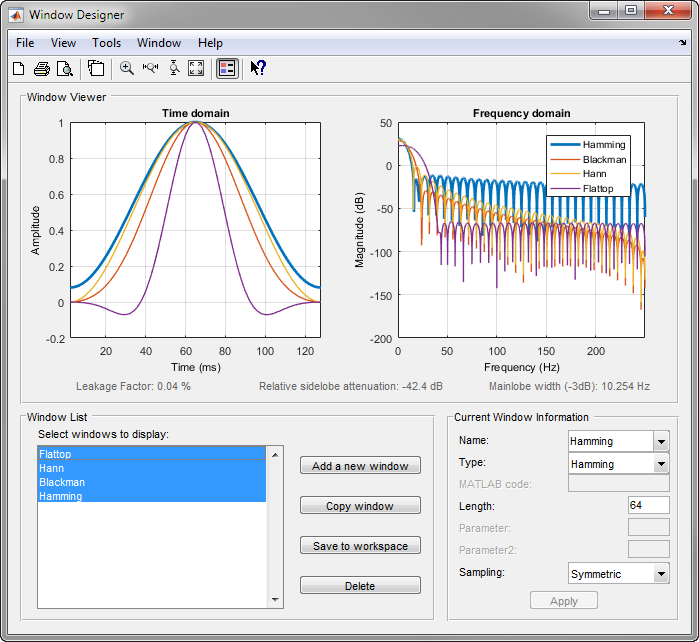## Generalized Cosine Windows

Blackman, flat top, Hamming, Hann, and rectangular windows are all special cases of the generalized cosine window. These windows are combinations of sinusoidal sequences with frequencies that are multiples of 2π/(N – 1), where N is the window length. One special case is the Blackman window:

```N = 128; A = 0.42; B = 0.5; C = 0.08; ind = (0:N-1)'*2*pi/(N-1); w = A - B*cos(ind) + C*cos(2*ind); ```

Changing the values of the constants `A`, `B`, and `C` in the previous expression generates different generalized cosine windows like the Hamming and Hann windows. Adding additional cosine terms of higher frequency generates the flat top window. The concept behind these windows is that by summing the individual terms to form the window, the low frequency peaks in the frequency domain combine in such a way as to decrease sidelobe height. This has the side effect of increasing the mainlobe width.

The Hamming and Hann windows are two-term generalized cosine windows, given by `A` = `0.54`, `B` = `0.46` for the Hamming and `A` = `0.5`, `B` = `0.5` for the Hann.

Note that the definition of the generalized cosine window shown in the earlier MATLAB® code yields zeros at samples 1 and `n` for `A` = `0.5` and `B` = `0.5`.

This Window Designer screen shot compares Blackman, Hamming, Hann, and Flat Top windows.